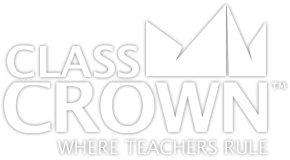## 3rd Grade Math Worksheets: Pack 1

Skills included in this pack:
• Dividing with number lines
• Multiplying with number lines
• Expressing multiplication
• Understanding multiplying by 10s
• Interpreting whole number division
• Interpreting arrays as multiplication
• Multiplying within 100, word problems
• Finding a missing fact in multiplication/division
• Finding missing values in multiplication & division equations
• Completing the associative property
• Finding associative property of multiplication
• Finding communicative property of multiplication
• Identifying distributive property of multiplication
• Using distributive property
• Division relative to multiplication
• Completing multiplication/division charts
• Multiplying within 100
• Dividing within 100
• Multiplying and dividing within 100
• Finding missing value in multiplication and division within 100
• Dividing in word problems (one digit quotient, no remainder)
• Dividing in word problems (one digit quotient with remainder)
• Solving 2-step word problems (add and then divide)
• Solving 2-step word problems (add and multiply)
• Determining which expression represents the solution to a word problem
• Solving 2-step word problems (multiply then add)
• Solving 2-step word problems (subtract & divide)
• Solving 2-step word problems (subtract and multiply)
• Determining rule in a function table
+ See More
Free Sample Pages Available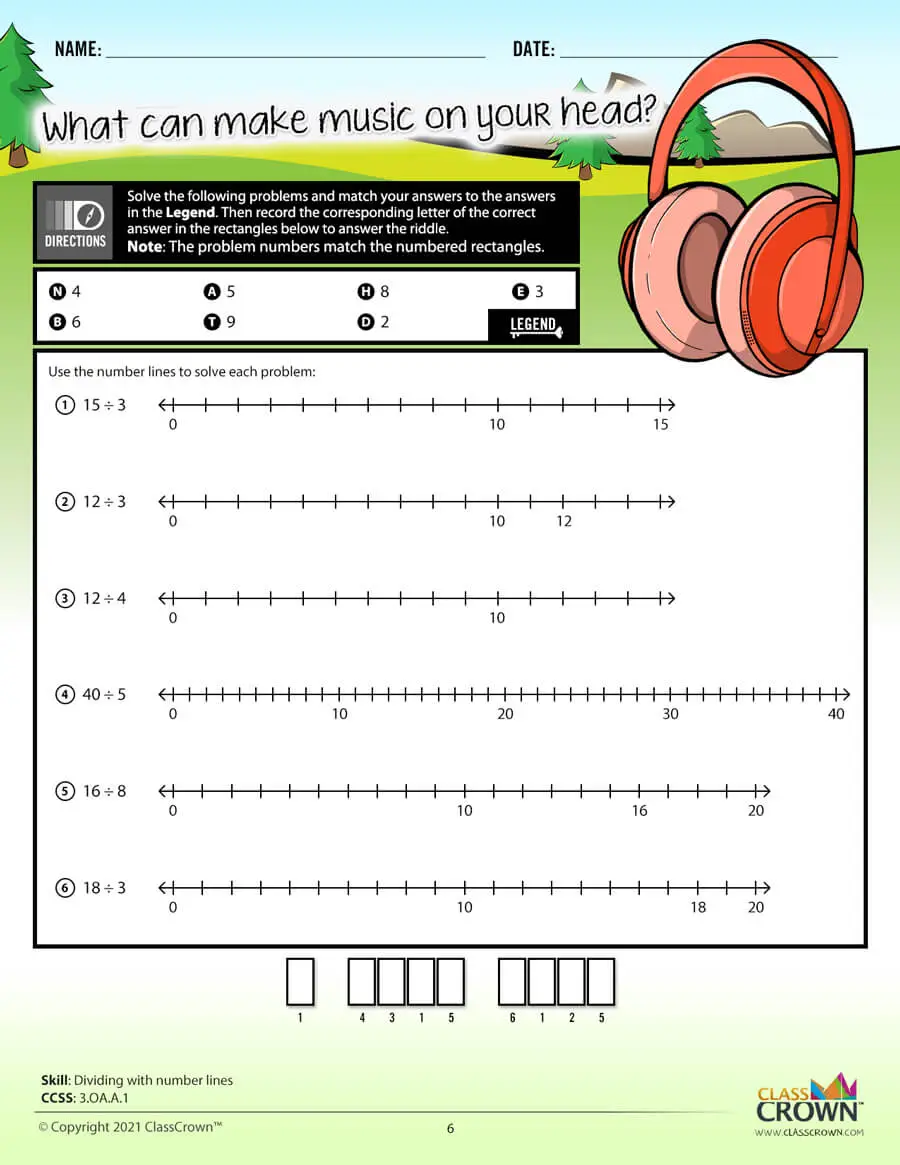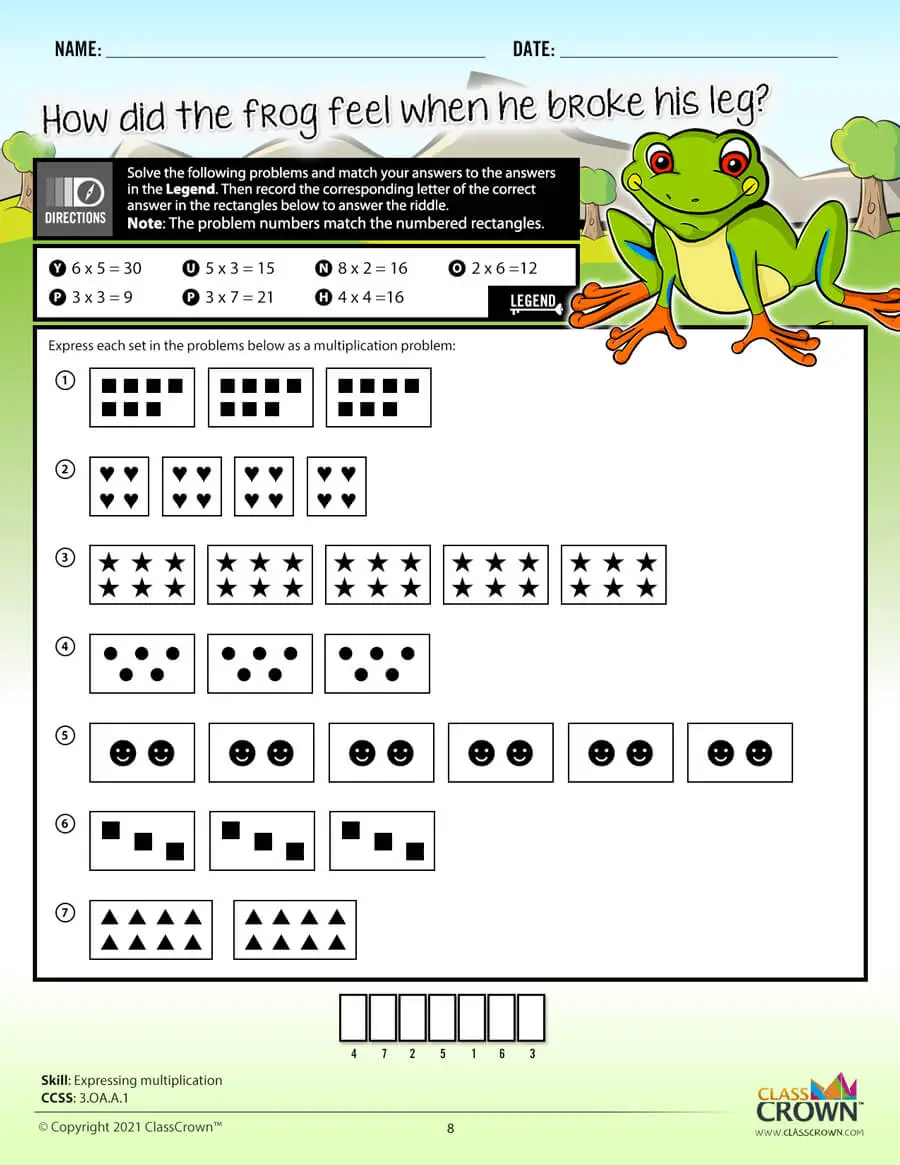## 3rd Grade Math Worksheets: Pack 2

Skills included in this pack:
• Estimating products
• Finding rounding amount
• Rounding to the nearest hundred with number lines
• Rounding to the nearest tens with number lines
• Rounding to tens and hundreds
• Subtracting within 1,000
• Adding and subtracting within 1,000
• Adding and subtracting within 1,000 with variables
• Subtracting across zero
• Multiplying multiples of 10
• Determining fractions (visual)
• Identifying fractions
• Writing fractions
• Subtracting fractions
• Finding fractions on a number line
• Finding equivalent fractions with number lines
• Comparing fractions
• Finding equivalent fractions
• Creating equivalent fractions with whole numbers
• Expressing whole numbers as fractions
• Expressing fractions as whole numbers
• Identify whole numbers as fractions
• Comparing fractions (same numerator and denominator)
• Comparing fractions (visual)
• Determining zero, half or whole
• Ordering fractions
• Comparing fractions
+ See More
Free Sample Pages Available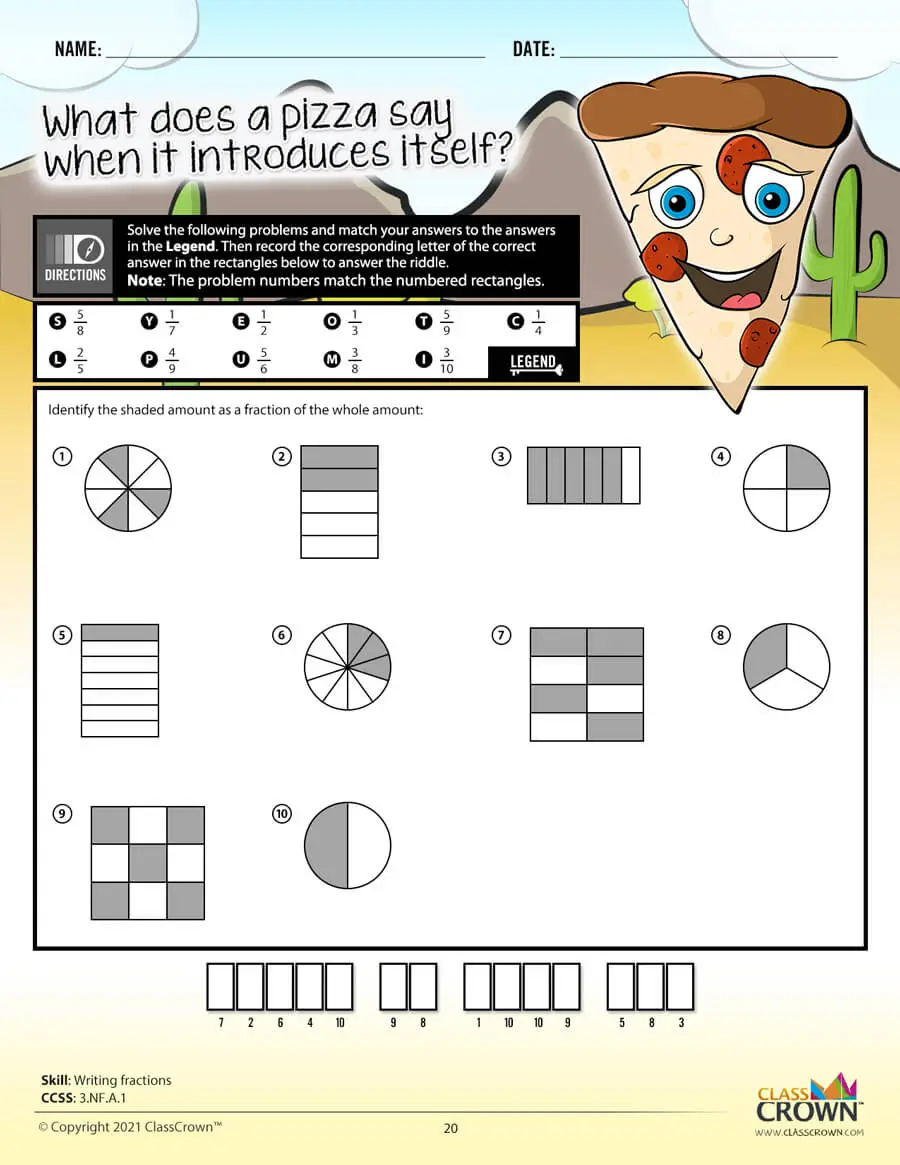## 3rd Grade Math Worksheets: Pack 3

Skills included in this pack:
• Reading analog clocks to 5-minute increments
• Reading analog clocks to the minute
• Finding elapsed time
• Finding elapsed time, word problems
• Determining ending time giver a starting time, word problems
• Converting times
• Convert times to only minutes
• Determining time given starting time
• Determining starting time given ending time
• Estimating capacity
• Estimating weight (metric)
• Matching bar graphs to tables
• Determining area with full blocks
• Determining area with full and half blocks
• Determining area with square units
• Finding area by tiling
• Finding area of rectangles by multiplying side lengths
• Determining rectilinear area
• Finding side length given area
• Finding side length given perimeter
• Identifying irregular shapes
• Identifying regular shapes
• Identifying regular and irregular shapes
• Identifying partitioned shapes
• Partitioning shapes
• Time, mixed review
• Area and perimeter, mixed review
+ See More
Free Sample Pages Available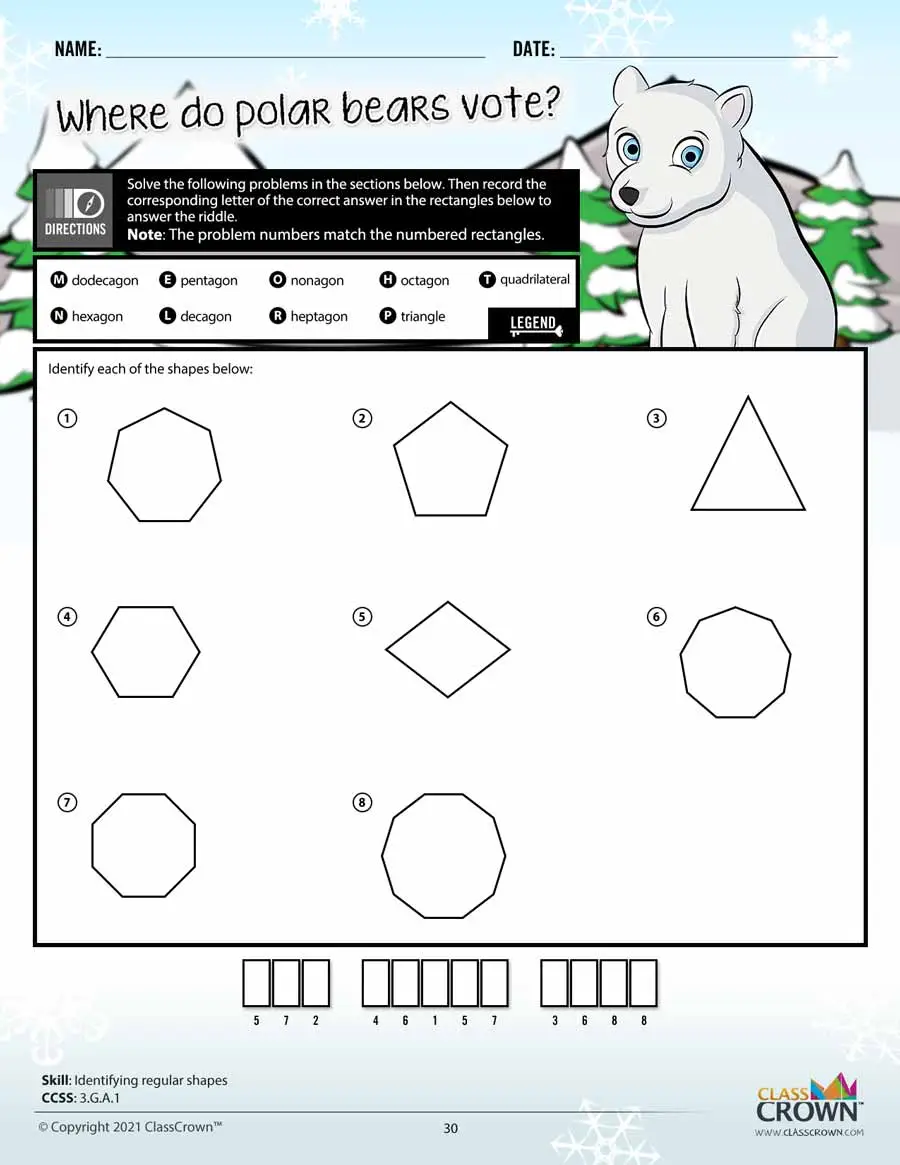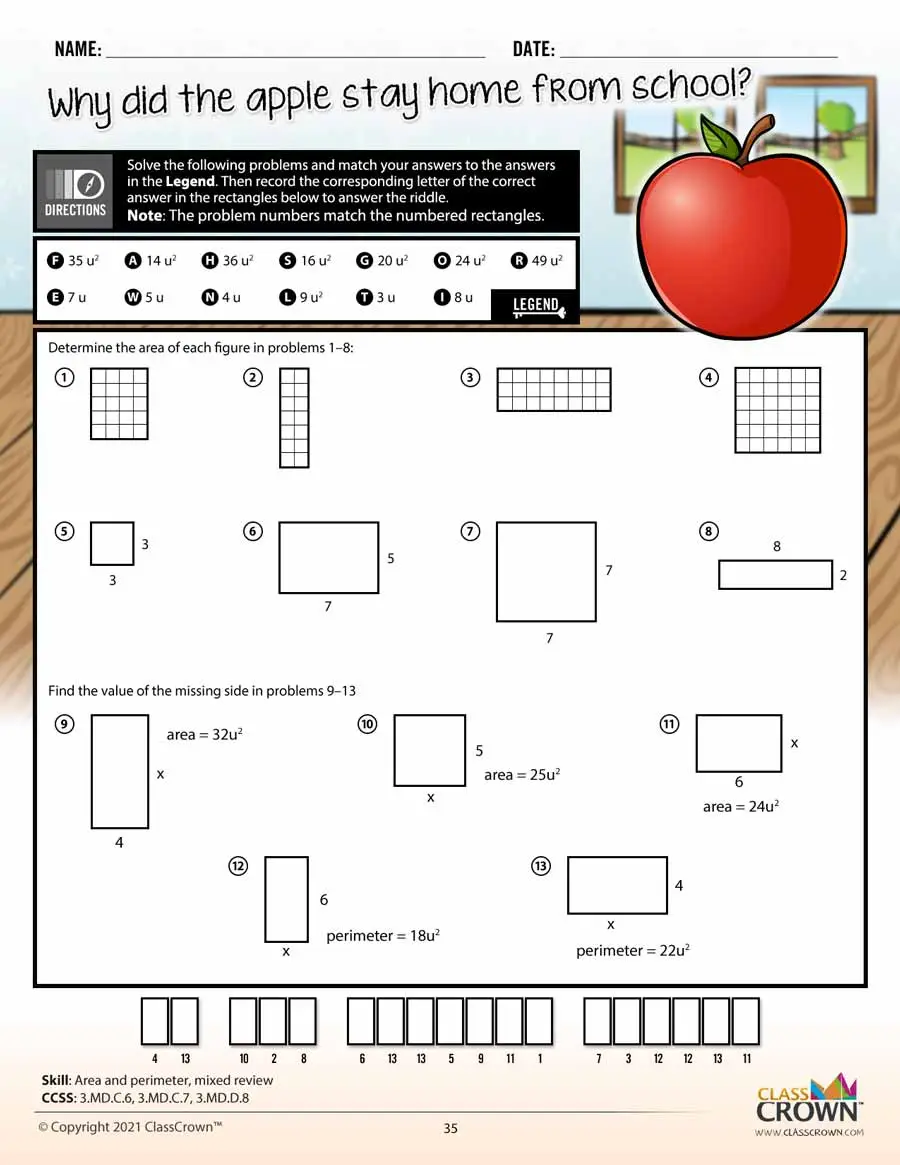Self Checking

Our math worksheets introduce a puzzle aspect to math, giving students immediate feedback as to whether or not they are solving problems correctly. If the answer to the riddle isn't spelled correctly, the student knows which problems he's made an error on.Fun Puzzle AspectImmediate Feedback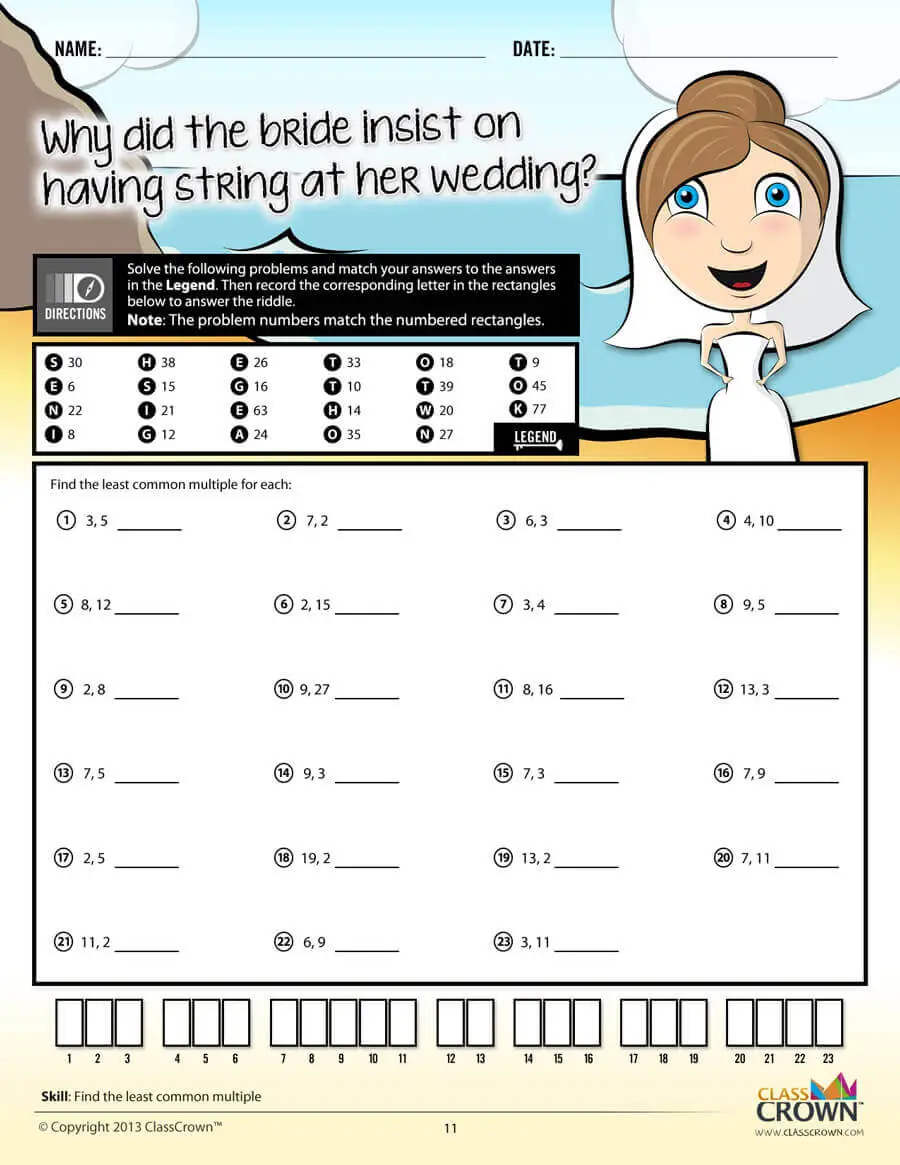Problem Solving Motivation

Each math worksheet contains a riddle that the student solves by completeing all the problems on the worksheet. This keeps kids motivated to complete each problem so that they can find the answer to the riddle.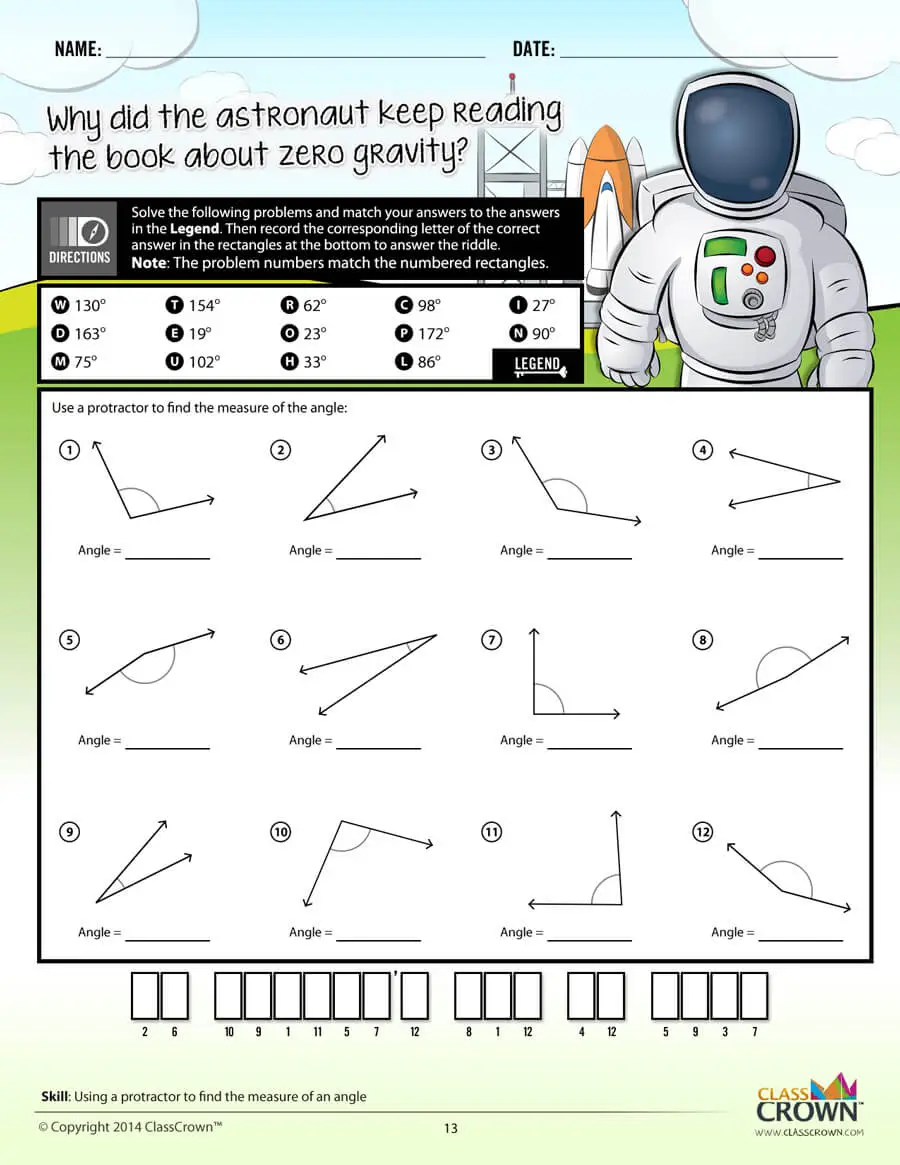Common Core Aligned

All our math worksheet packs are designed with Common Core in mind. That way you don’t have to worry about whether your math ciriculum is aligned or not when you incorpoate ClassCrown Riddle-Me-Worksheets in your lesson plans.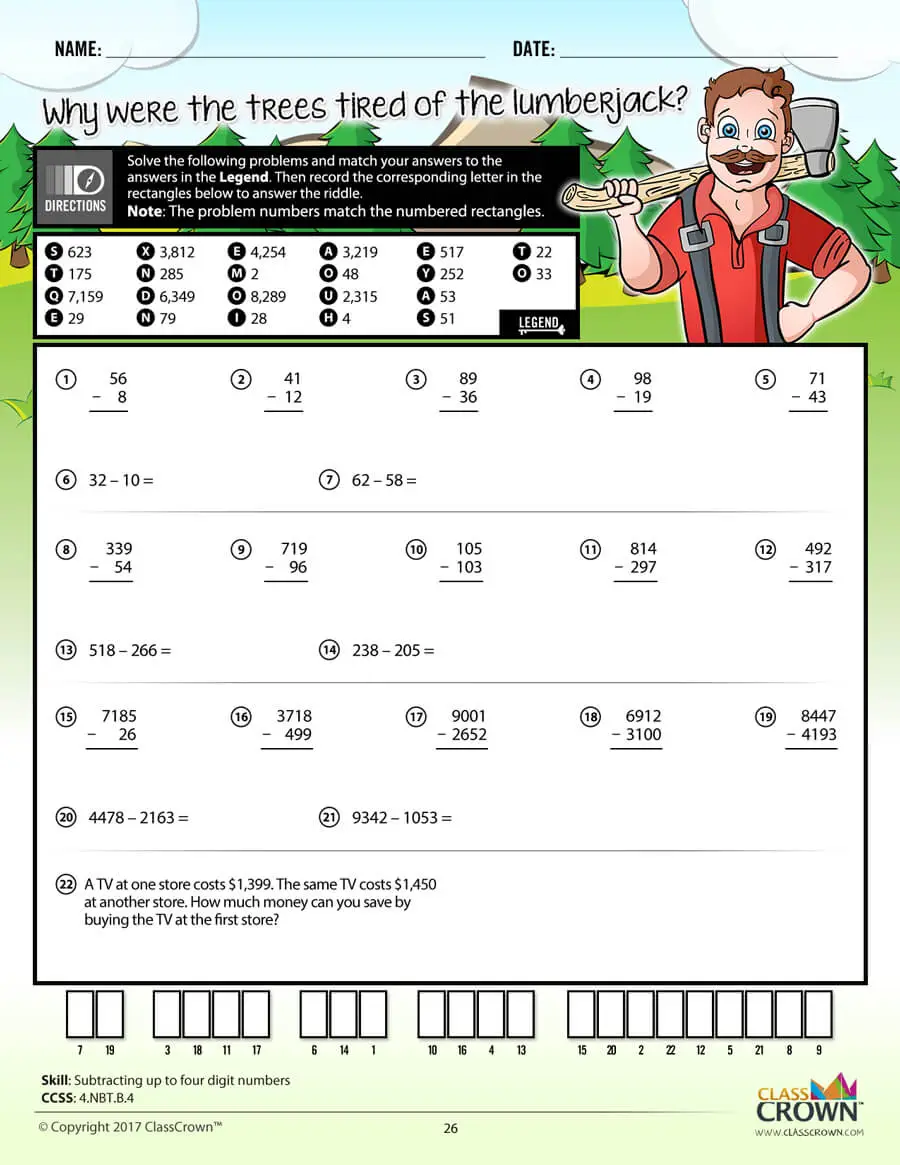High Quality Design

Each page of our math worksheets has been produced in high resolution at 144 dpi and designed in full, vibrant color for maximum quality. They look stunning whether you are printing in color or black and white.High Resolution
(144 dpi)Stunning Color & Clarity